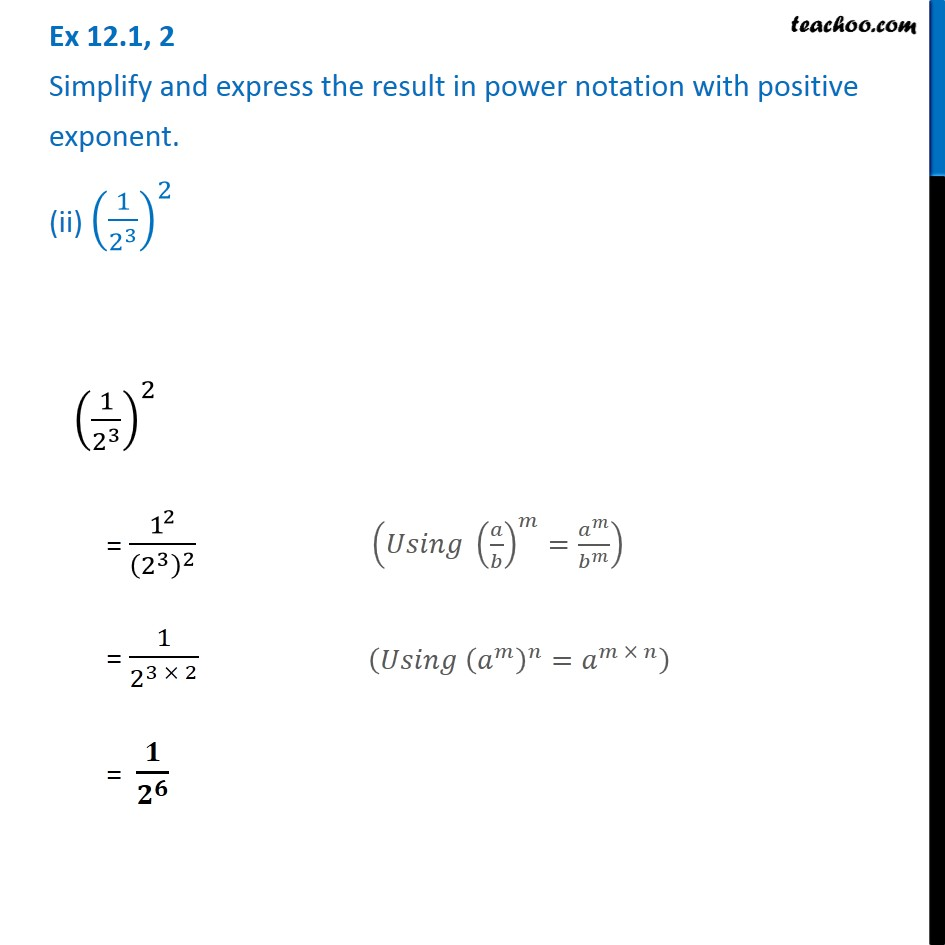Ex 12.1

Chapter 12 Class 8 Exponents and Powers
Serial order wise### Transcript

Ex 12.1, 2 Simplify and express the result in power notation with positive exponent. (ii) (1/2^3 )^2 (1/2^3 )^2 = 1^2/(2^3 )^2 = 1/2^(3 × 2) = 𝟏/𝟐^𝟔 (𝑈𝑠𝑖𝑛𝑔 (𝑎/𝑏)^𝑚=𝑎^𝑚/𝑏^𝑚 ) (𝑈𝑠𝑖𝑛𝑔 (𝑎^𝑚 )^𝑛=𝑎^(𝑚 × 𝑛) )